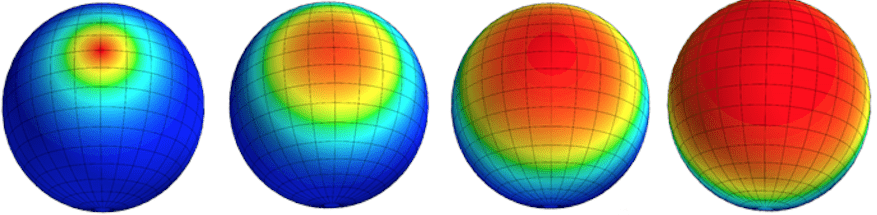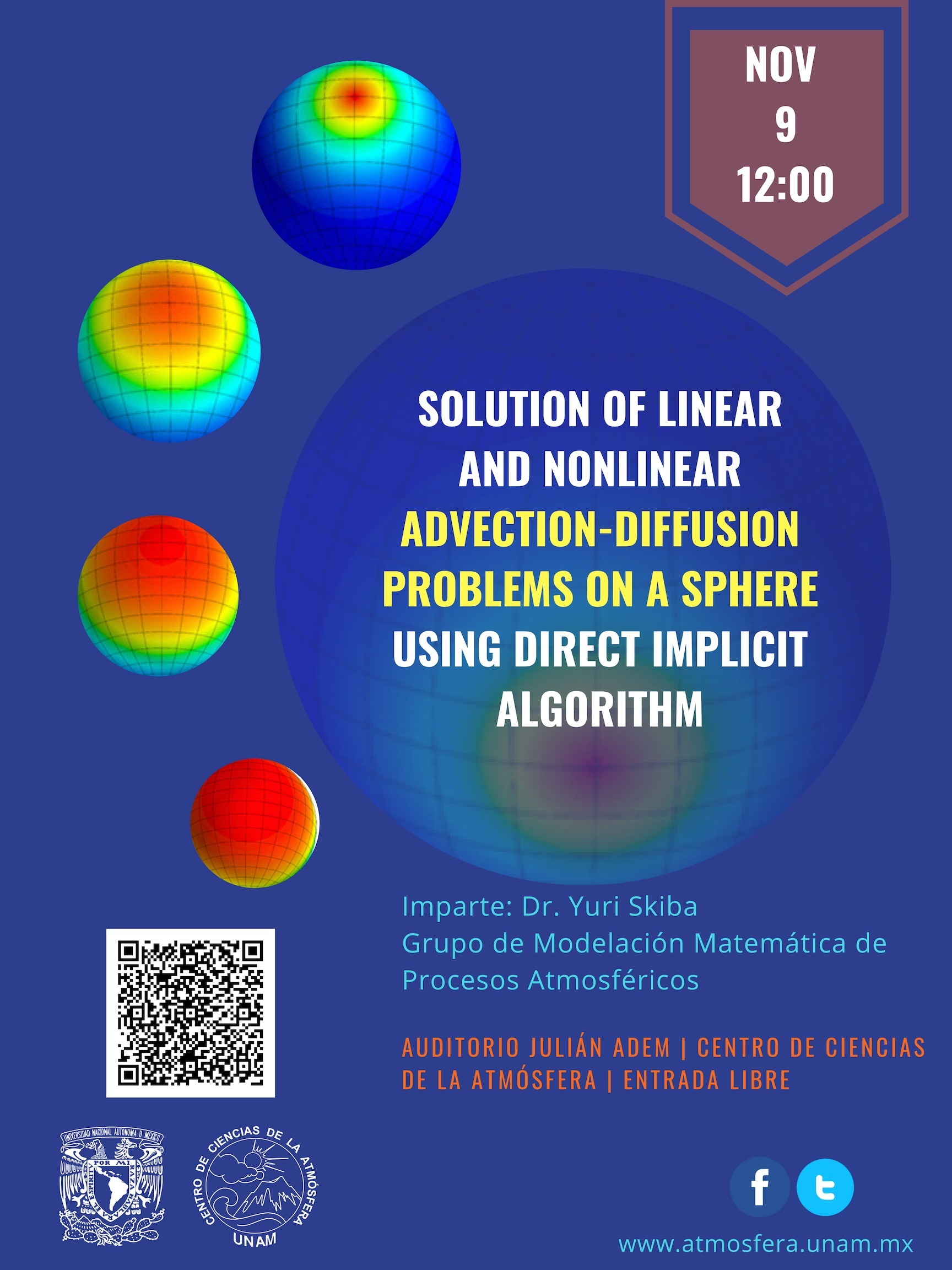# Solution of Linear and Nonlinear Advection-Diffusion Problems on a Sphere Using Direct Implicit Algorithm

Imparte: Dr. Yuri Skiba

Modelación Matemática de Procesos Atmosféricos

The numerical algorithm proposed in  is applied for solving linear and nonlinear advection-diffusion problems on the surface of a sphere. The velocity field on a sphere is assumed to be known and non-divergent. The discretization of advection-diffusion equation in space is carried out with the help of the finite volume method, and the Gauss theorem is applied to each grid cell. For the discretization in time, the symmetrized double-cycle componentwise splitting method and the Crank-Nicolson scheme are used. The one-dimensional periodic problems arising at splitting in the longitudinal direction are solved with Sherman-Morrison’s formula and Thomas’s algorithm. The one-dimensional problems arising at splitting in the latitudinal direction are solved by the bordering method that requires a prior determination of the solution at the poles. The resulting linear systems have tridiagonal matrices and are solved by Thomas’s algorithm. The algorithm is of second order approximation in space and time. It is implicit, unconditionally stable, direct (without iterations) and rapid in realization. The theoretical results obtained in  are confirmed numerically by simulating various linear and nonlinear advection-diffusion processes. The results show high accuracy and efficiency of the method that correctly describes the advection-diffusion processes and balance of mass of substance in the forced and dissipative discrete system. In addition, it conserves the total mass and -norm of the solution in the absence of external forcing and dissipation. As it was mentioned in , the new method can also be applied to some elliptic problems and adjoint advection-diffusion problems on a sphere.

 Y.N. Skiba, A non-iterative implicit algorithm for the solution of advection-diffusion equation on a sphere, International Journal for Numerical Methods in Fluids 78 (2015), 257-282.Nov 09 2018

### Horario

12:00 pm - 1:00 pm

### Precio